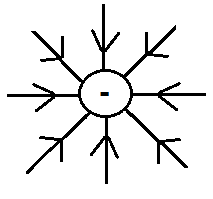﻿ Electric Force Calculator ﻿# Electric Force CalculatorEnter the Electric field, E: N/C Enter the Charge, Q: pC nC µC mC C

0.0000000000 N

This electric force calculator calculates the electric force of an object based on the electric field of the object and the amount of charge that the object has.

To use this calculator, a user just has to enter in the electric field, E, and the charge, Q, and then click the 'Submit' button, and the resultant answer will automatically be computed and shown. The electric field must be entered in in unit newtons per coulombs (N/C). The charge of the object can be entered in picocoulombs, nanocoulombs, microcoulombs, millicoulombs, or coulombs, but will be converted to coulombs for calculation. The resultant answer, the electric force, will be calculated in unit newtons (N).

The electric force of a charge or charged item is directly proportional to the magnitude of its electric field and net charge. This means that the greater the magnitude of the electric field, the greater the electric force which the object will exert. Conversely the lesser the magnitude of the electric field, the lesser the electric force. Electric charge follows the same direct relationship. The greater the charge, the greater the electric force which the charged object exerts. While the lesser the charge, the lesser the force.

Electric force is an important calculation in the field of electronics because electric force exists in any type electronic components which emanate electric fields. One such popular component are capacitors. Capacitors are devices which storage electric charge on their parallel plates. These charges emanate an electric field, which in turn exerts an electric force on any nearby items. Calculating the electric force quantifies how greatly it pulls or pushes (depending on the type of charge present) another charged item nearby. Thus, calculating the electric force can be important and critical in many different types of electronic circuits.

Example Calculation

If an object has an electric field, E, of 10N/C and a charge of 2C, what is the electric force of this object?

So based on the values of the parameters, we use the equation F=EQ and substitute in the values into the equation.

F= (10N/C)(2C)= 20N

Related Resources

﻿